# pvigier's blog

computer science, programming and other ideas

## Terrain generation in Simulopolis

Hi!

Today I will describe the generator of terrains I made for Simulopolis.

Here are some screenshots of terrains from the game.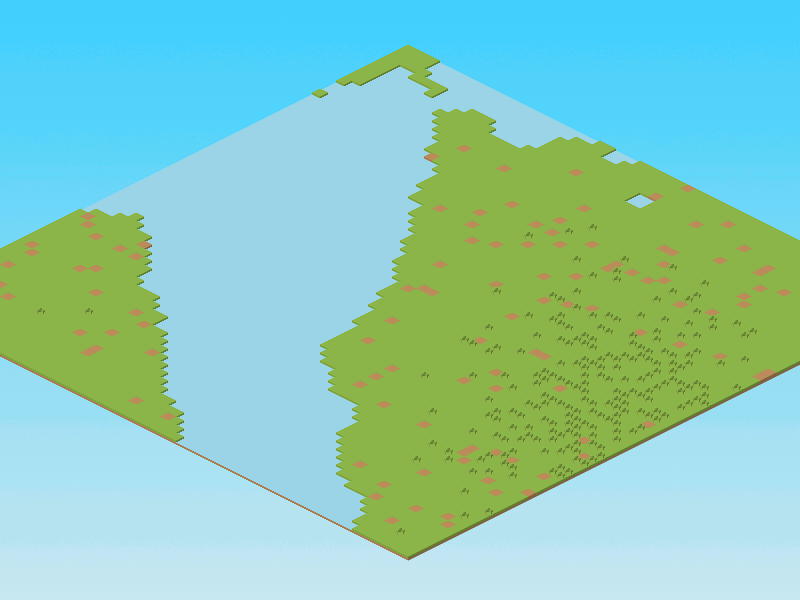In this article, I will not describe the C++ code used in the game as it is verbose and unnecessarily complicated. Instead, I will use the Python code I used to design the generator.

Indeed, I found much more pleasant to use Python for prototyping as it is really fast to write something which works, there are good libraries and we do not have to wait for compilation every time we make a change. It is especially true for procedural content generation where we have to iterate a lot before obtaining something decent.

The code I will present is the exact translation in Python of what is currently implemented in the game. It is based on the implementation of Perlin noise I described previously in this article. As usual, you can find the whole project on github.

# Grass

We will store the terrain in a 2D array. And we will map integers to the different types of tiles like that:

• 0: water
• 1: grass
• 2: trees
• 3: dirt

Let us define a 2D array of size 64x64 initialized to 1:

n = 64
grid = np.ones((n, n), dtype=np.int32)


At this step, we just have a completely green and boring map: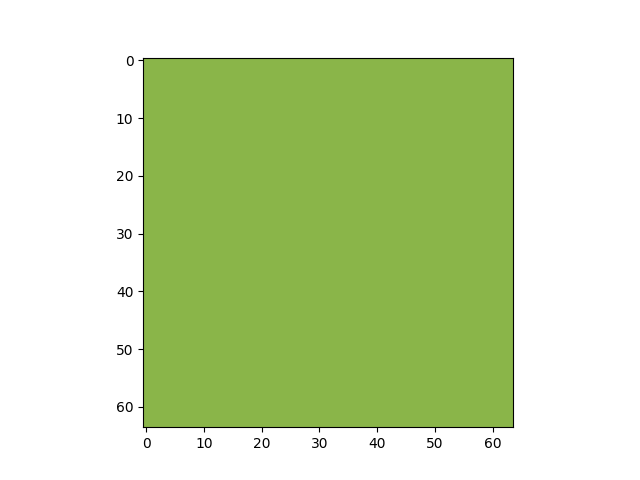# Let there be noise

Then let us generate some fractal noise with six octaves using the function I made last time:

noise = generate_fractal_noise_2d((n, n), (1, 1), 6)
noise = (noise - noise.min()) / (noise.max() - noise.min())


Notice that the values of the noise are normalized between 0 and 1.

Here what it looks like: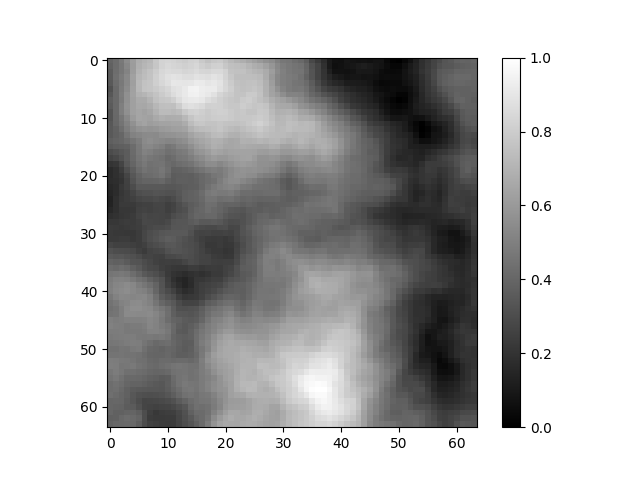# Water

The rule to add water is very simple. If the noise is below a threshold then the tile becomes water otherwise it stays green:

threshold = 0.3
grid[noise < threshold] = 0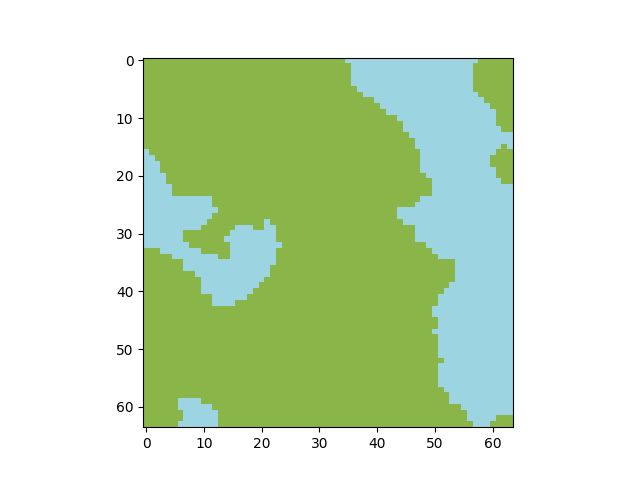# Trees

The rule for generating trees is more complicated. I wanted to put trees far from water, in the hills. Thus, I reuse the previous noise texture and interpret it as altitude.

At the tiles that are not water, the noise is between threshold and 1. I normalize this value between 0 and 1 again by using this formula: (noise - threshold) / (1 - threshold).

I could again take a threshold and set all the tiles where the threshold is exceeded to be trees. But it would not be very aesthetic. I would like to have some holes inside the forest.

Instead, I generate a sort of potential using the noise like that:

potential = ((noise - threshold) / (1 - threshold))**4 * 0.7


Here is this potential for the current map: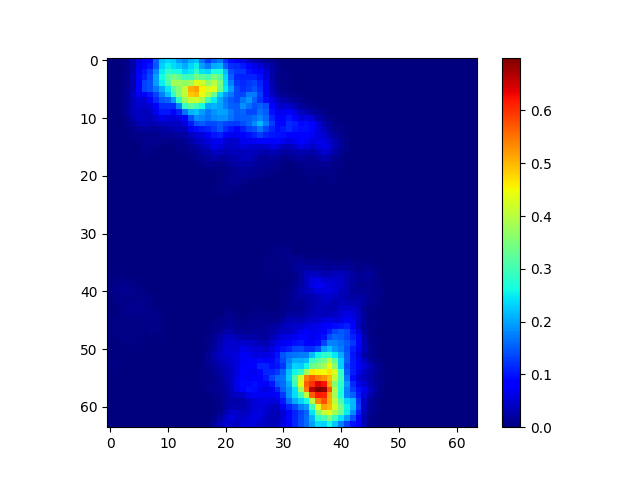Finally, for each grass tile we generate a uniform random number. And if this number is smaller than the potential then the tile becomes a tree:

mask = (noise > threshold) * (np.random.rand(n, n) < potential)


You can see two parameters in the formula for the potential: 4 and 0.7. The first one controls how fast the potential decreases when we approach water and the second one controls how it is easy to become a tree.

The map with trees: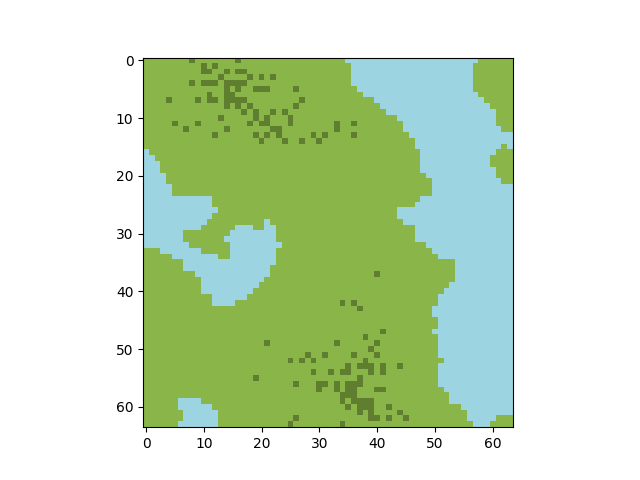# Dirt

The last step is to add some dirt. The rule is very simple, I transform a grass tile into dirt with probability 0.05:

mask = (grid == 1) * (np.random.rand(n, n) < 0.05)


Here is the final result: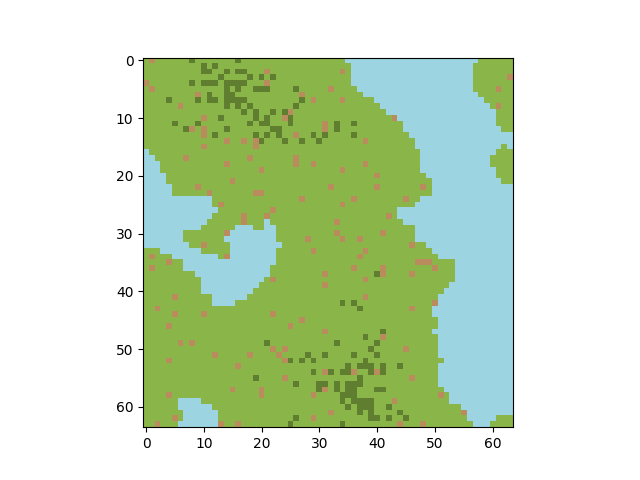The whole process is pretty simple but I find the results decent. I hope you find it interesting to see how my generator is working.

See you!

If you are interested in my adventures during the development of Vagabond, you can follow me on Twitter.

Tags: pcg simulopolis

Subscribe to the newsletter if you do not want to miss any new article: